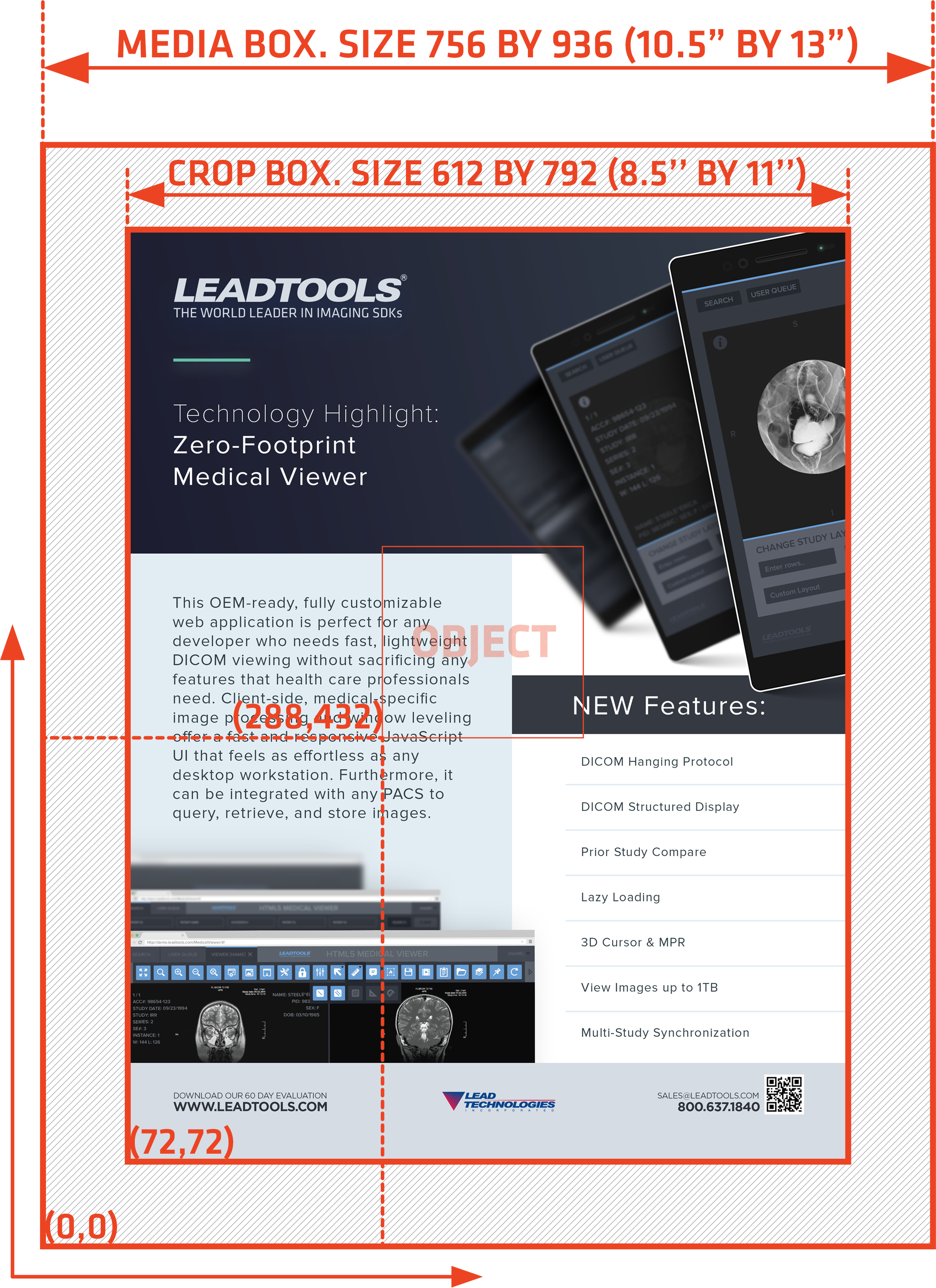﻿ PDF Coordinate System | Raster, Medical, Document Help

# PDF Coordinate System

PDF documents have the following properties:

• The origin of the document is at the bottom-left corner. The x-axis extends to the right and the y-axis extends upward.
• All locations and sizes are stored as logical values in PDF units. One PDF unit is equivalent to a DTP point (1/72").
• PDF documents do not have a resolution. To convert a PDF unit to a physical value such as pixels, the external value for the resolution must be provided.

For example, a typical PDF document may have a page width and height of 612 by 792 units. Hence:

• The size in inches is 8.5" by 11" (Obtained by dividing the width of 612 and the height of 792 by 72)
• An object located at point 288, 432 in the PDF has a distance of 4" by 6" from the bottom-left corner of the page (Also obtained by dividing each coordinate of the location point by 72)

The following image shows this typical PDF document and an object:PDF documents contain a media box and optionally a crop box. If the PDF document does not define a crop box, then it is the same as the media box. The media box defines the width and height of the page, while the crop box defines the viewable/printable area. PDF viewers typically do not show objects that are located outside of the crop box and report the size of the page to be the width and height of the media box. So when a PDF viewer such as Adobe Acrobat Reader opens the document shown above, it will report the page size to be 10.5" by 13", even though the crop box size is 8.5" by 11". Object locations are always calculated based on the media box, regardless of the existence and position of the crop box.

Physical values such as pixels are needed when a PDF document is rendered to the screen. To convert from logical to physical units, a resolution must be provided. Resolution is the number of dots (pixels) per inch to use when converting logical to physical values. In the example above, if a resolution of 150 is used, then the size of the page in pixels is 1575 by 1950 (obtained by multiplying 10.5" and 13" by 150). It is also desirable to obtain the converted values in top-left coordinates when converting from PDF units (logical) to pixels (physical), since most rendering systems such as `System.Drawing` and `System.Windows.Media` use the top-left corner of the window as the origin.

PDF documents do not contain a resolution: it is up to the user to specify resolution when rendering the document. High resolution values will render the document with greater detail at the expense of using more system resources. Typical resolution values are 72, 96, 150, 200, 300, and 600.

If the document shown in the image above is rendered with a resolution of 150, then the object will be located at the following pixel values:

````Pixel X value = (288 / 72) * 150 = 600 `
`Pixel Y value = ((936 - 432) / 72) * 150 = 1050 ````

Where 936 is the height of the page in DTP units, 432 is the Y coordinate in DTP units, 72 is the number of DTP units in an inch, and 150 is the resolution

Notice that when the Y-pixel value is calculated, the Y-coordinate value is subtracted from the height of the page, thus converting the coordinates from bottom-left to top-left origin.

When using the `Leadtools.Pdf.PDFFile` or `Leadtools.Pdf.PDFDocument` classes of `Leadtools.Pdf`, LEADTOOLS reads the values from the PDF document without conversion. The following values are read in PDF units (1/72"):

The `PDFDocument.Resolution` property holds the resolution value to use when converting between logical (PDF units) and physical (pixels). This property value can be changed at any time.

The helper methods `PDFDocumentPage.ConvertPoint` and `PDFDocumentPage.ConvertRect` can be used to convert from any unit to another. These methods require a `Leadtools.Pdf.PDFCoordinateType` enumeration member for the source and destination units.

The following code converts a point (sourcePoint) from PDF units to inches and pixels:

````PDFPoint destPointInches = pdfDocumentPage.ConvertPoint(PDFCoordinateType.Pdf, PDFCoordinateType.Inch, sourcePoint); `
`PDFPoint destPointPixels = pdfDocumentPage.ConvertPoint(PDFCoordinateType.Pdf, PDFCoordinateType.Pixel, sourcePoint); ````

The following code converts a rectangle (sourceRect) from PDF units to inches and pixels:

````PDFRect destRectInches = pdfDocumentPage.ConvertRect(PDFCoordinateType.Pdf, PDFCoordinateType.Inch, sourceRect); `
`PDFRect destRectPixels = pdfDocumentPage.ConvertRect(PDFCoordinateType.Pdf, PDFCoordinateType.Pixel, sourceRect); ````

The following code converts the points and rectangles back to PDF units:

````sourcePoint = pdfDocumentPage.ConvertPoint(PDFCoordinateType.Inch, PDFCoordinateType.Pdf, destPointInches); `
`sourcePoint = pdfDocumentPage.ConvertPoint(PDFCoordinateType.Pixel, PDFCoordinateType.Pdf, destPointPixels); `
`sourceRect = pdfDocumentPage.ConvertRect(PDFCoordinateType.Inch, PDFCoordinateType.Pdf, destRectInches); `
`sourceRect = pdfDocumentPage.ConvertRect(PDFCoordinateType.Pixel, PDFCoordinateType.Pdf, destRectPixels); ````

The `Leadtools.Pdf.PDFDocumentPage` object always uses the value of the `PDFDocument.Resolution` property when converting between logical and physical coordinates.